Next: About this document Up: Solids of Revolution Previous: Surface Area

## Exercises

1. For each function below,
1. Plot its surface of revolution.
2. Find the volume of the solid of revolution about the x-axis.
3. Find the surface area of the surface of revolution about the x-axis.
•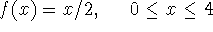•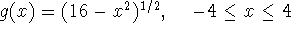•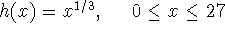2. Now generalize the results from Exercise 1 by taking advantage of Maple's symbolic capabilites and find the volume and surface area for:
1.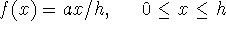about the x-axis
2.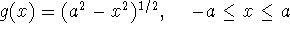about the x-axis
3.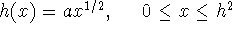about the x-axis
(Your answers in each case may have parameters a and/or h in them. Also, results for parts a and b should already be known to you!)
3. Consider the set of all points satisfying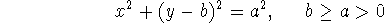1. Describe the set of points.
2. If this curve is rotated about the x-axis, describe the resulting surface and solid of revolution.
3. What is its surface area?
4. What is its volume?
5. Why is the conditionimportant geometrically?

Sean O Anderson
Tue Dec 3 12:06:23 EST 1996